20 Rule of Thumb: the Economic half-life Time

Already in the measured values for the investment banking industry a certain half-life can be seen. So we ask ourselves what such dynamic effects are a result of and whether there is a way to estimate such times, at least roughly. Thus we consider the following highly simplified model of a closed economy: An economy has the economic performance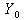given to a start time. Whereas a lot of moneyis delivered.is slightly larger than, so that all things can be paid for with money in circulation, as well as a general reserve assetcan be accumulated: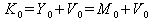(20.1)

With the growth of the economy now is growing the capital stock. Thus we can write(20.2)

It is considered that arises more money with the economic growth41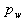, and the inflation rate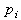becomes effective and, finally, the deposits with the banks earn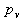of interest. We compute, as always, on an annual basis, therefore the time factor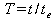is the number of years. We are now following the question: At what junctureis the capital stock greater than the sum of tradable goods? So mathematically, the inequality:(20.3) ?

Now, the relative share of capital stock at all levels of the economy, goods and assets, results from the ratio of capital stock to total national wealth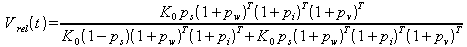(20.4)

and to reduce the fracture results in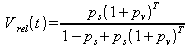(20.5).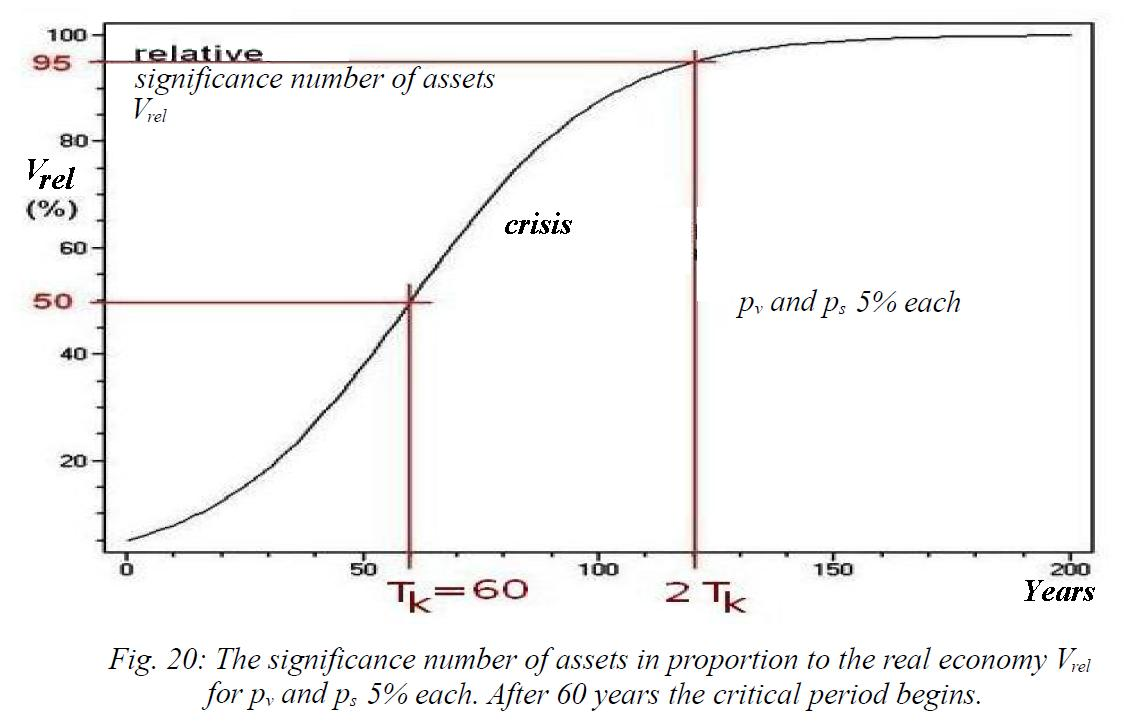This gives a dimensionless value that is called the "significance number" of capital in an economy. When this value gets greater than 0.5 = 50%, so there will be a transition, after which the meaning of production falls behind the financial sector . This equation can now be easily resolved to the critical time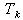, as in the case of equality holds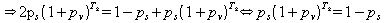(20.6)

and thus after a short algebra we get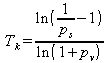(20.7).

As is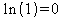, one can also see that the critical timegoes to infinite foror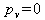, which corresponds to a stable system. Typical values for the savings rate and interest rate are about 10% and 5% in the FRG. This gives a critical time of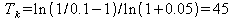years.

For moderate values, about 7% and 3%, the corresponding time is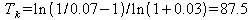years,

which is already more than two generations. The critical time of a monetary economy depends so much on the return on investment: With every percentage point it drops considerably. Somewhat less strongly is the influence of the savings rate. With only 1% for each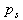and, the critical time is more than 400 years. An increase in interest rates to 10% results in a lifetime of only a few decades. An increase in the savings rate alone to 10% reduced but this time not much, it is then still almost 300 years. At moderate values ofandthe critical period is therefore from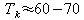years.The rule of thumb for the critical period can be reduced to a simple rule because of the shallow curve of the natural logarithm. Asandare significantly smaller than one and commute to values of about 5%=0.05, one can roughly approximate: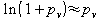and(20.8).

So that we can remember as a "raw rule of thumb" for the average lifetimes of capital driven economies:(20.9).

With average interest rate on all assets of about 5%, we obtain for example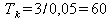years     (20.10)

magnitude as for the estimation of the saturation level.